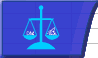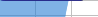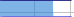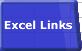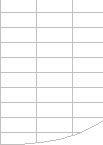# Formula Evaluation CircumstancesSmart Recalculation Evaluation Circumstances Calculation Process Dependency Trees Controlling Calculation "CALCULATE" in Status Bar Calculation Methods Calculating from VBA Volatile Functions & Actions User-Defined Functions Repetitive Calculation Version Timing Comparison### Circumstances causing a formula to be evaluated.

A formula or part of a formula will be immediately evaluated whenever:

• It is entered or changed.
• It is entered or changed via the Function Wizard.
• It is entered as an argument in the Function Wizard.
• It is selected in the formula bar and F9 is pressed (press Esc to Undo and revert to the formula).

A formula in a conditional format will be evaluated at each recalculation or screen repaint of the cell containing the conditional format (conditional formats are volatile).

A formula will be flagged as uncalculated whenever it refers to (depends on) a cell, formula, name or value that has been

• entered.
• changed.
• is in an Autofiltered list and the criteria pulldown has been activated.
• is flagged as uncalculated.

Certain actions will also unexpectedly flag a formula as uncalculated or trigger a recalculation: see volatile actions.

A formula that is flagged as uncalculated will be evaluated whenever the Range, Worksheet, Workbook or Excel instance that contains it is calculated or recalculated.

Note that a recalculation may also occur when opening a workbook in Automatic mode or when saving a workbook in Manual Mode with the Calculate before Save option. (See Controlling Calculation)

### Circumstances causing a name to be evaluated.

The circumstances causing a name to be evaluated are not the same as a formula in a cell.

• A name seems to only be evaluated when a formula that refers to it is evaluated.
• A name is evaluated every time a formula that refers to it is evaluated, so that using a name in multiple formulae can cause the name to be evaluated multiple times.
• Names that are NOT referred to by any formula are not calculated even by a full calculation (Ctrl/Alt/F9).© 2001-2020 Decision Models Legal Disclaimer Privacy Statement